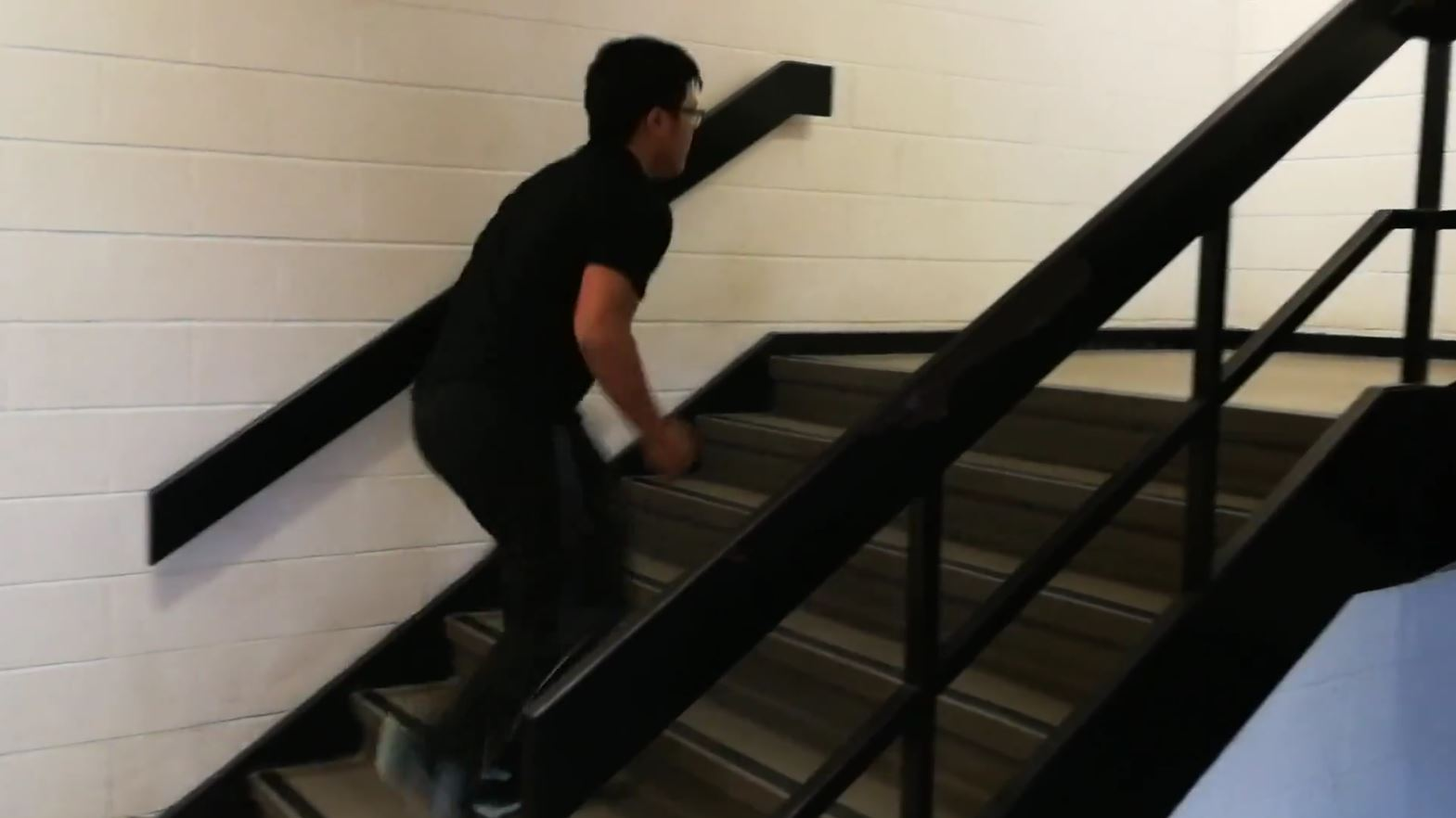## Power!

Today, we were introduced to the concept of power!

During the class lesson and our group investigation of power, we tested everyone’s individual leg power from walking up stairs to help us in our understanding of power. Here’s what I learned:

1) Putting together what has been learned in the past few days, work represents the change in total energy within the system; when you do work, you are putting in energy into a system (whether that be by lifting it or exerting a force). Note that when you are comparing two moments in time, whatever happens in between is irrelevant to finding how much work has been done. So, when you move a box by certain distance horizontally by pushing it from rest and then bringing back to rest, you are in fact doing no work at all. The box did not change in kinetic energy as it is still at rest, and the box did not change in gravitational potential energy as it did not move up or down.

W = ΔE

2) Power is the ratio of amount of work done (W) to the time interval (Δt) that it takes to do the work: P = W/Δt. As such, the most powerful machines are ones that transfer the most mechanical energy in the least amount of time.

P = W/Δt = ΔE/Δt

3) The derived SI unit of power is the watt (W). This is not to be confused with work (W). Since power is work divided by the time interval, it can also be expressed in terms of joules per second. If we take the base units of joules and apply it to the watt, we can express power as kgm2s-3.

1 W = 1 Js-1 = 1 kgm2s-3

Comparing this new information with my previous understanding of power, I have a few questions:

1) In electrical circuits, we find the power is the product of voltage and current. How does this relate to power in mechanical applications? Does this mean that a typical phone charger that outputs 5 W (5 V 1 A) is able to exert 5 N of force on a 1 kg object over 1 m in 1 s?

2) What is the typical power output of a human? Does our power output depend heavily on the task?

After finding everyone’s individual power output, we collected all of our data and found some intriguing correlations!

Power was inversely correlated with the time taken to climb the stairs. This is fairly obvious given the equation P = W/Δt.

However, power showed an extremely weak positive correlation with the mass of the person. Even though people with higher mass do more work by climbing the stairs, it seems that the time taken to climb the stairs varied so much compared to the mass of the people that mass was made insignificant.

#### After Unit Reflection

##### Understanding Energy - Power

In this blog post, I showed my understanding of power in physics: the rate at which work is done. I learned that power is the ratio of the amount of work done to the time interval, and can be expressed in different forms: watts (W), joules per second (Js-1), and kilogram metres squared per second cubed (kgm2s-3).